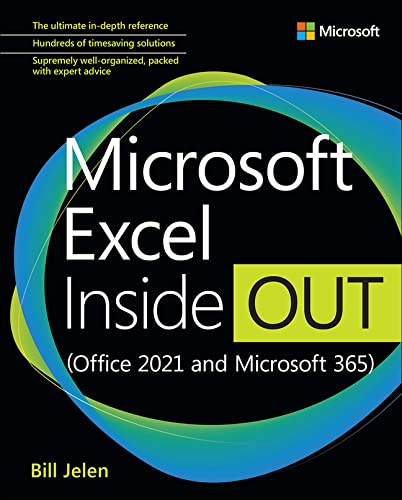# Microsoft Excel Inside Out

Rating: 4.2 out of 5 5 reviews
• Length: 848 pages
• Edition: 1
• Publisher:
• Publication Date: 2021-12-16
• ISBN-10: 0137559534
• ISBN-13: 9780137559534

## Book Description

Conquer Microsoft Excel — from the inside out!

Dive into Microsoft Excel — and really put your spreadsheet expertise to work. This supremely organized reference packs hundreds of timesaving solutions, tips, and workarounds — all you need to make the most of Excel’s most powerful tools for analyzing data and making better decisions.

Renowned Excel expert Bill Jelen offers a complete tour of Excel in Office 365, from efficient interface customizations to advanced analysis, visualizations, and dashboards. Discover how experts tackle today’s key tasks — and challenge yourself to new levels of mastery. Topics include:

• Customizing the Excel workspace
• Best practices for designing and managing worksheets
• Creating formulas and functions
• Performing statistical, what-if, and other data analysis
• Core to advanced charting techniques
• Using graphics and sparklines
• Managing databases and tables
• Automating Excel with macros and custom functions
• Collaborating in Excel online, in the cloud, and more
• Extending Excel

``````Part I: The Excel interface
Chapter 1. What’s new in Microsoft 365 Excel
Chapter 2. Using the Excel interface
Chapter 3. Customizing Excel
Chapter 4. Keyboard shortcuts

Part II: Calculating with Excel
Chapter 5. Understanding formulas
Chapter 6. Controlling formulas
Chapter 7. Understanding functions
Chapter 8. Using everyday functions: math, date and time, and text functions
Chapter 9. Using powerful functions: logical, lookup, and database functions
Chapter 10. Using names, LET, LAMBDA, and Data Types in Excel
Chapter 11. Connecting worksheets and workbooks
Chapter 12. Dynamic array formulas and names in Excel

Part III: Data analysis with Excel
Chapter 13. Transforming data with Power Query
Chapter 14. Summarizing data using subtotals or filter
Chapter 15. Using pivot tables to analyze data
Chapter 16. Using slicers and filtering a pivot table
Chapter 17. Mashing up data with Power Pivot
Chapter 18. Using What-If, Scenario Manager, Goal Seek, and Solver
Chapter 19. Automating repetitive functions using VBA macros
Chapter 20. More tips and tricks for Excel

Part IV: Excel visuals
Chapter 21. Formatting worksheets
Chapter 22. Using data visualizations and conditional formatting
Chapter 23. Graphing data using Excel charts
Chapter 24. Using 3D Maps
Chapter 25. Using sparklines
Chapter 26. Formatting spreadsheets for presentation
Chapter 27. Printing
Chapter 28. Collaborating in Excel

Part V: Appendixes
Appendix A. Excel functions
Appendix B. Excel functions with DAX or Power Query equivalents